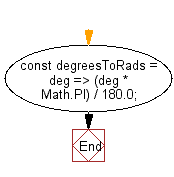# JavaScript: Convert an given angle from degrees to radians

## JavaScript fundamental (ES6 Syntax): Exercise-246 with Solution

Write a JavaScript program to convert angles from degrees to radians.

• Use Math.PI and the degree to radian formula to convert the angle from degrees to radians.

Sample Solution:

JavaScript Code:

``````//#Source https://bit.ly/2neWfJ2
const degreesToRads = deg => (deg * Math.PI) / 180.0;
```
```

Sample Output:

```1.5707963267948966
0.5235987755982988
```

Pictorial Presentation:Flowchart:Live Demo:

See the Pen javascript-basic-exercise-246-1 by w3resource (@w3resource) on CodePen.

Improve this sample solution and post your code through Disqus

What is the difficulty level of this exercise?

Test your Programming skills with w3resource's quiz.

﻿